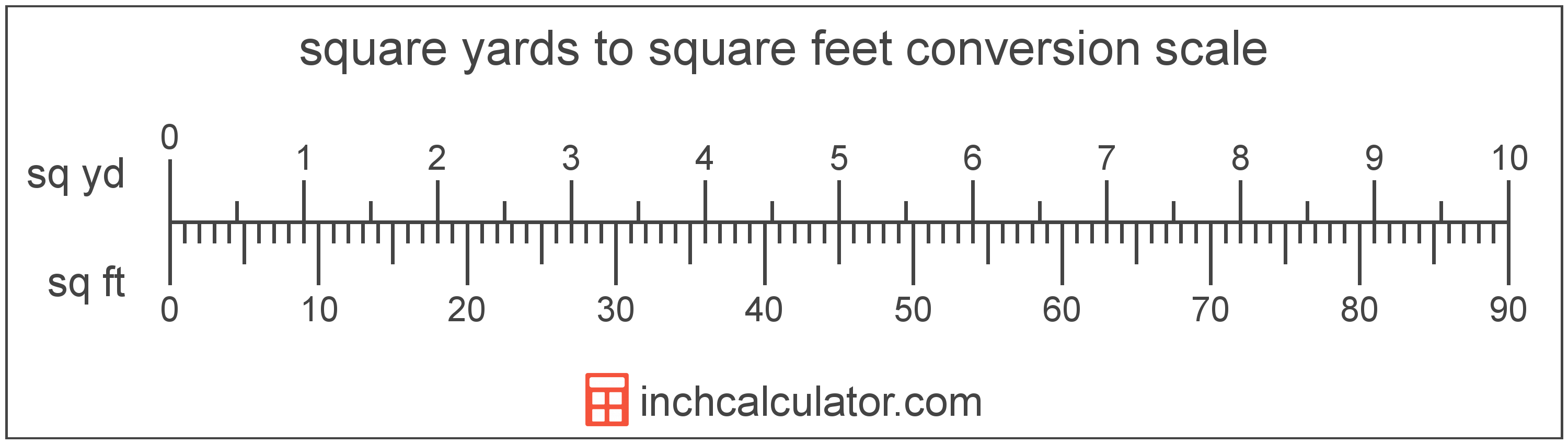# Square Yards to Square Feet Converter

Enter the area in square yards below to get the value converted to square feet.

(find square yards)
Results in Square Feet:1 sq yd = 9 sq ft

Do you want to convert square feet to square yards?

## How to Convert Square Yards to Square Feet

To convert a measurement in square yards to a measurement in square feet, multiply the area by the following conversion ratio: 9 square feet/square yard.

Since one square yard is equal to 9 square feet, you can use this simple formula to convert:

square feet = square yards × 9

The area in square feet is equal to the area in square yards multiplied by 9.

For example, here's how to convert 5 square yards to square feet using the formula above.
square feet = (5 sq yd × 9) = 45 sq ft### How Many Square Feet Are in a Square Yard?

There are 9 square feet in a square yard, which is why we use this value in the formula above.

1 sq yd = 9 sq ft

## What is a Square Yard?

One square yard is equivalent to the area of a square with sides that are each 1 yard in length. One square yard is roughly equal to 9 square feet or 0.836127 square meters.

The square yard is a US customary and imperial unit of area. A square yard is sometimes also referred to as a square yd. Square yards can be abbreviated as sq yd, and are also sometimes abbreviated as yd². For example, 1 square yard can be written as 1 sq yd or 1 yd².

You can use a square yards calculator to calculate the area of a space if you know its dimensions.

## What is a Square Foot?

One square foot is equivalent to the area of a square with sides that are each 1 foot in length. One square foot is equal to 144 square inches or 0.092903 square meters .

The square foot is a US customary and imperial unit of area. A square foot is sometimes also referred to as a square ft. Square feet can be abbreviated as sq ft, and are also sometimes abbreviated as ft². For example, 1 square foot can be written as 1 sq ft or 1 ft².

You can use a square footage calculator to calculate the area of a space if you know its dimensions.

## Square Yard to Square Foot Conversion Table

Table showing various square yard measurements converted to square feet.
Square Yards Square Feet
1 sq yd 9 sq ft
2 sq yd 18 sq ft
3 sq yd 27 sq ft
4 sq yd 36 sq ft
5 sq yd 45 sq ft
6 sq yd 54 sq ft
7 sq yd 63 sq ft
8 sq yd 72 sq ft
9 sq yd 81 sq ft
10 sq yd 90 sq ft
11 sq yd 99 sq ft
12 sq yd 108 sq ft
13 sq yd 117 sq ft
14 sq yd 126 sq ft
15 sq yd 135 sq ft
16 sq yd 144 sq ft
17 sq yd 153 sq ft
18 sq yd 162 sq ft
19 sq yd 171 sq ft
20 sq yd 180 sq ft
21 sq yd 189 sq ft
22 sq yd 198 sq ft
23 sq yd 207 sq ft
24 sq yd 216 sq ft
25 sq yd 225 sq ft
26 sq yd 234 sq ft
27 sq yd 243 sq ft
28 sq yd 252 sq ft
29 sq yd 261 sq ft
30 sq yd 270 sq ft
31 sq yd 279 sq ft
32 sq yd 288 sq ft
33 sq yd 297 sq ft
34 sq yd 306 sq ft
35 sq yd 315 sq ft
36 sq yd 324 sq ft
37 sq yd 333 sq ft
38 sq yd 342 sq ft
39 sq yd 351 sq ft
40 sq yd 360 sq ft

## References

1. Merriam-Webster, square foot, https://www.merriam-webster.com/dictionary/square%20foot